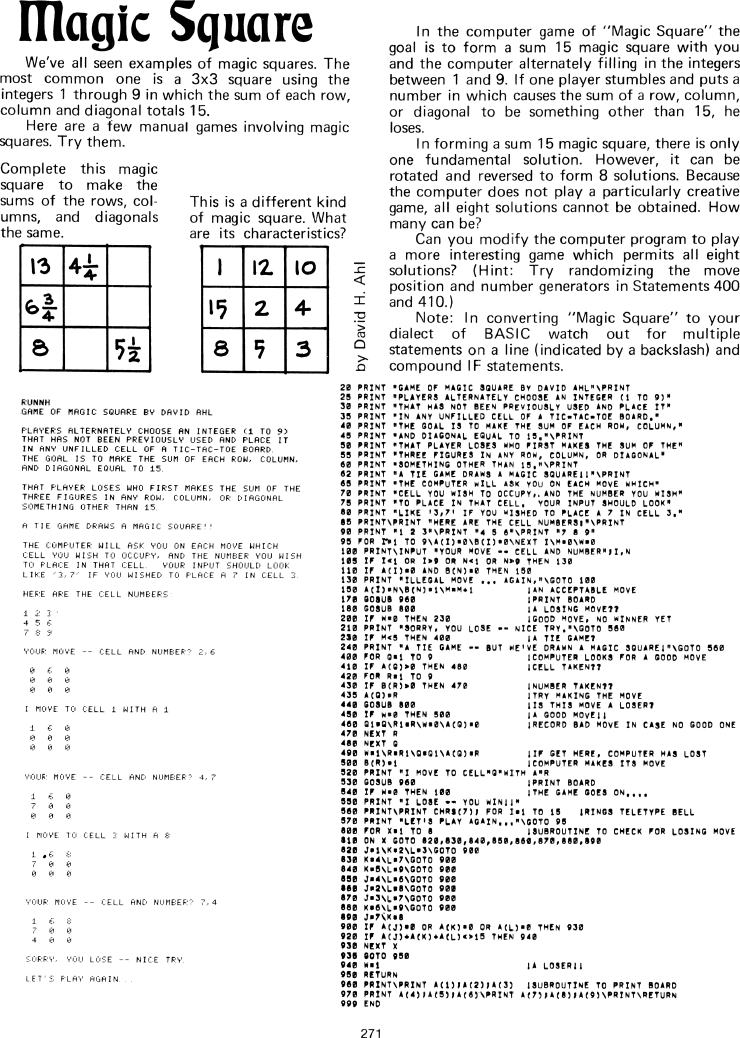The Best of Creative Computing Volume 1 (published 1976)

 `Page 271 << PREVIOUS >> NEXT Jump to page: Go to contents Go to thumbnails`

Magic Square (addition, BASIC computer game, BASIC program listing, sample run)```Magic Square

We've all seen examples of magic squares. The most common one is a 3x3 square
using the integers 1 through 9 in which the sum of each row, column and diagonal
totals 15.

Here are a few manual games involving magic squares. Try them.

Complete this magic square to make the sums of the rows, columns, and diagonals
the same.

[image]13 4 1/4 6 3/4 8 5 1/2

This is a different kind of magic square. What are its characteristics?

[image]1 12 10 15 2 4 8 5 3

In the computer game of "Magic Square" the goal is to form a sum 15 magic square
with you and the computer alternately filling in the integers between 1 and 9.
lf one player stumbles and puts a number in which causes the sum of a row,
column, or diagonal to be something other than 15, he loses.

ln forming a sum 15 magic square, there is only one fundamental solution.
However, it can be rotated and reversed to form 8 solutions. Because the
computer does not play a particularly creative game, all eight solutions cannot
be obtained. How many can be?

Can you modify the computer program to play a more interesting game which
permits all eight solutions? (Hint: Try randomizing the move position and number
generators in Statements 400 and 410.)

Note: In converting "Magic Square" to your dialect of BASIC watch out for
multiple statements on a line (indicated by a backslash) and compound IF
statements.

by David h. Ahl

RUNNH

GAME OF MAGIC SQUARE BY DAVID AHL

PLAYERS ALTERNATELY CHOOSE AN INTEGER (1 TO 9) THAT HAS NOT BEEN PREVIOUSLY USED
AND PLACE IT IN ANY UNFILLED CELL OF A TIC-TAC-TOE BOARD. THE GOAL IS TO MAKE
THE SUM OF EACH ROW, COLUMN, AND DIAGONAL EQUAL 15.

THAT PLAYER LOSES WHO FIRST MAKES THE SUM OF THE THREE FIGURES IN ANY ROW,
COLUMN, OR DIAGONAL SOMETHING OTHER THAN 15.

A TIE GAME DRAWS A MAGIC SQUARE!!

THE COMPUTER WILL ASK YOU ON EACH MOVE WHICH CELL YOU WISH TO OCCUPY, AND THE
NUMBER YOU WISH TO PLACE IN THAT CELL. YOUR INPUT SHOULD LOOK LIKE '3,7' IF YOU
WISHED TO PLACE A 7 IN CELL 3.

HERE ARE THE CELL NUMBERS:

123

456

789

YOUR MOVE -- CELL AND NUMBER? 2,6

0 6 0

0 0 0

0 0 0

I MOVE TO CELL 1 WITH A 1

1 6 0

0 0 0

0 0 0

YOUR MOVE -- CELL AND NUMBER? 4,7

1 6 0

7 0 0

0 0 0

I MOVE TO CELL 3 WITH A 8

1 6 8

7 0 0

0 0 0

YOUR MOVE -- CELL AND NUMBER? 7,4

1 6 8

7 0 0

4 0 0

SORRY, YOU LOSE -- NICE TRY

LET'S PLAY AGAIN

20 PRINT "GAME OF MAGIC SQUARE BY DAVID AHL"\PRINT
25 PRINT "PLAYERS ALTERNATELY CHOOSE AN INTEGER (1 TO 9)"

30 PRINT "THAT HAS NOT BEEN PREVIOUSLY USED AND PLACE IT"

35 PRINT "IN ANY UNFILLED CELL OF A TIC-TAC-TOE BOARD."

40 PRINT "THE GOAL IS TO MAKE THE SUM OF EACH ROW, COLUMN,"

45 PRINT "AND DIAGONAL EQUAL 15."\PRINT
50 PRINT "THAT PLAYER LOSES WHO FIRST MAKES THE SUM OF THE"

55 PRINT "THREE FIGURES IN ANY ROW, COLUMN, OR DIAGONAL"

60 PRINT "SOMETHING OTHER THAN 15."\PRINT
62 PRINT "A TIE GAME DRAWS A MAGIC SQUARE!!"\PRINT
65 PRINT "THE COMPUTER WILL ASK YOU ON EACH MOVE WHICH"

70 PRINT "CELL YOU WISH TO OCCUPY, AND THE NUMBER YOU WISH"

75 PRINT "TO PLACE IN THAT CELL. YOUR INPUT SHOULD LOOK"

80 PRINT "LIKE '3,7' IF YOU WISHED TO PLACE A 7 IN CELL 3."

85 PRINT\PRINT "HERE ARE THE CELL NUMBERS:"\PRINT
90 PRINT "1 2 3"\PRINT "4 5 6"\PRINT "7 8 9"

95 FOR I=1 TO 9\A(I)=0\5(I)=0\NEXT I\H=0\W=0
100 PRINT\INPUT "YOUR MOVE -- CELL AND NUMBER";I,N
105 IF I<1 OR I>9 OR N<1 OR N>9 THEN 130
110 IF A(I)=0 AND B(N)=0 THEN 150
130 PRINT "ILLEGAL MOVE ... AGAIN,"\GOTO 100
150 A(I)=N\B(N)=1\M=M+1         |AN ACCEPTABLE MOVE
170 GOSUB 960                   |PRINT BOARD
180 GOSUB 800                   |A LOSING MOVE??

200 IF W=0 THEN 230             |GOOD MOVE, NO WINNER YET
210 PRINT "SORRY, YOU LOSE -- NICE TRY."\GOTO 560
230 IF H<5 THEN 400             |A TIE GAME?

240 PRINT "A TIE GAME -- BUT WE'VE DRAWN A MAGIC SQUARE!"\GOTO 560
400 FOR Q=1 TO 9                |COMPUTER LOOKS FOR A GOOD MOVE
410 IF A(Q)>0 THEN 480          |CELL TAKEN??

420 FOR R=1 TO 9
430 IF B(R)>0 THEN 470          |NUMBER TAKEN??

435 A(Q)=R                      |TRY MAKING THE MOVE
440 GOSUB 800                   |IS THIS MOVE A LOSER?

450 IF W=0 THEN 500             |A GOOD MOVE!!

460 Q1=Q\R1=R\W=0\A(Q)=0        |RECORD BAD MOVE IN CASE NO GOOD ONE
470 NEXT R
480 NEXT Q
490 WRI\R=R1\Q=Q1\A(Q)=R        |IF GET HERE, COMPUTER HAS LOST
500 B(R)=1                      |COMPUTER MAKES ITS MOVE
520 PRINT "I MOVE TO CELL"Q"WITH A "R
530 GOSUB 960                   |PRINT BOARD
540 IF W=0 THEN 100             |THE GAME GOES ON....

550 PRINT "I LOSE -- YOU WIN!!"

560 PRINT\PRINT CHRS(7); FOR I=1 TO 15    |RINGS TELETYPE BELL
570 PRINT "LET'S PLAY AGAIN..."\GOTO 95
800 FOR X=1 TO 8
810 ON X GOTO 820,830,840,850,860,870,880,890
820 J=1\K=2\L=3\GOTO 900
830 K=4\L=7\GOTO 900
840 K=5\L=9\GOTO 900
850 J=4\L=6\GOTO 900
860 J=2\L=8\GOTO 900
870 J=3\L=7\GOTO 900
880 K=6\L=9\GOTO 900
890 J=7\K=8
900 IF A(J)=0 OR A(K)=0 OR A(L)=O THEN 930
920 IF A(J)+A(K)+A(L)<>15 THEN 940
930 NEXT X
935 GOTO 950
940 W=1                          |A LOSER!!

950 RETURN
960 PRINT\PRINT A(1);A(2);A(3)   |SUBROUTINE TO PRINT BOARD
970 PRINT A(4);A(5);A(6)\PRINT A(7);A(8);A(9)\PRINT\RETURN
999 END

271Page 271        << PREVIOUS        >> NEXT        Jump to page:                Go to contents        Go to thumbnails

<!--